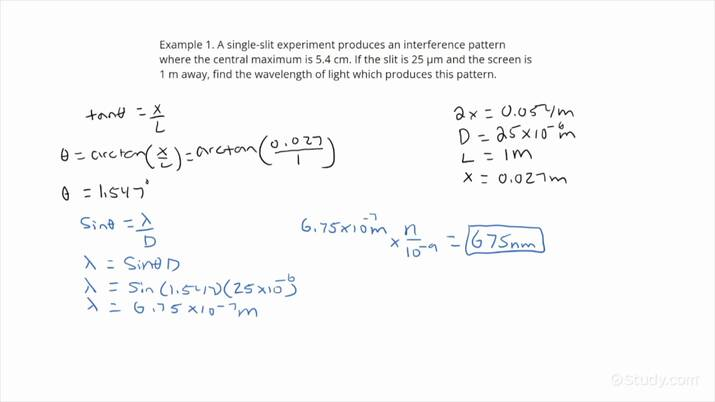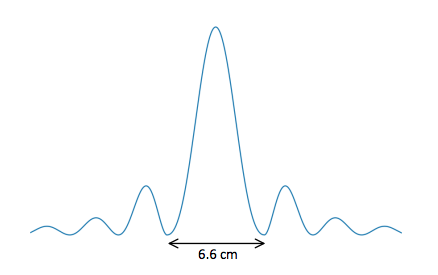# How to Find the Wavelength of Light in a Single Slit Experiment Using the Spacing in the Interference PatternAn error occurred trying to load this video.

Try refreshing the page, or contact customer support.

• 00:12 Find the wavelength of…
• 02:56 Find the wavelength of…
Leah Simon, Christian Miller
• Instructors
Leah Simon

Leah Simon has a Ph. D. in Astronomy from the University of Florida and a Bachelor of Arts degree in Physics from Macalester College. She has taught college physics and astronomy for over 10 years and has worked for several years as a lesson writer for high school and college physics laboratory courses.

•
Christian Miller

Christian Miller has tutored college physics and taught microbiology laboratories as a teaching assistant for two years. Christian completed a Bachelor of Science in Biomedical Sciences from Sam Houston State University.

## How to Find the Wavelength of Light in a Single Slit Experiment Using the Spacing in the Interference Pattern

Step 1: Determine the width of the slit D, the width of the central maximum of the interference pattern 2x or the distance between two neighboring minima not surrounding the central maximum in the interference pattern x, and the distance from the slit to the screen L.

Step 2: Calculate the angle {eq}\theta {/eq} using the equation:

{eq}\tan\theta=\frac{x}{L} {/eq}.

Step 3: Use {eq}\theta {/eq} from the previous step to solve the equation

{eq}\sin\theta=\frac{\lambda}{D} {/eq}

for the wavelength {eq}\lambda {/eq}.

Step 4: Check that the units match and the wavelength is in the range of visible light.

## What is Single Slit Experiment and its Interference Pattern?

Single Slit Experiment: Monochromatic light passes through a single narrow slit of width D and illuminates a screen a distance L away where {eq}L>>D {/eq}. Because light is a wave, the single slit bends or diffracts the light near the edges of the slit so that light passing through the center of the slit interferes with light passing through the edges of the slit, creating a diffraction pattern on the screen.

Interference or Diffraction Pattern: The pattern of bright and dark fringes produced by the diffraction of light through a single slit. In the single slit experiment, the central maximum is much brighter than the maxima on either side and is twice as wide as the maxima on either side. The minima to one side of the central maximum are evenly spaced a distance x apart (for small-angle approximation).

Diffraction Pattern Equation: The diffraction minima occur when the path difference between light rays passing through the top and bottom of the slit is an integer number of the wavelength. The path difference depends on the angle at which the light rays enter the slit and is describe by the equation below:

{eq}D\sin\theta=m\lambda {/eq}, where m = 1, 2, 3, .... m = 0 corresponds to the central maximum, not a minimum. This equation can be combined with the geometry of the slit, screen, and diffraction pattern to relate the diffraction pattern to the wavelength of light {eq}\lambda {/eq} and slit width D.

Let's practice using the interference pattern in a single slit experiment to find the wavelength of light in the following two examples. In the first example, we will use the width of the central maximum in the interference pattern. In the second example, we will use the spacing of the minima in the interference pattern.

## Examples of Finding the Wavelength of Light in a Single Slit Experiment Using the Interference Pattern

Example 1

Find the wavelength of light in nanometers incident on a 10-micron slit that produces the interference pattern shown on a screen 50 cm away.Step 1: Determine the width of the slit D, the width of the central maximum of the interference pattern 2x, and the distance from the slit to the screen L.

The slit width and the distance from the slit to the screen are given in the problem. The width of the central maximum of the interference pattern is shown in the figure.

{eq}D=10~\mu \text{m} = 1\times 10^{-5}~\text{m} {/eq}

L = 50 cm = 0.50 m

2x = 6.6 cm; x = 3.3 cm = 0.033 m

Note that each length is given in different units, but in order to do any calculations, we need to convert all lengths to the same units. For simplicity, we convert all lengths to meters before performing any calculations.

Step 2: Calculate the angle {eq}\theta {/eq} using the equation:

{eq}\tan\theta=\frac{x}{L} {/eq}.

Plug in the values from the previous step to solve for {eq}\theta {/eq}.

{eq}\theta = \arctan{\frac{x}{L}}=\arctan{\frac{0.033~\text{m}}{0.50~\text{m}}}=3.776^\circ {/eq}

Step 3: Use {eq}\theta {/eq} from the previous step to solve the equation

{eq}\sin\theta=\frac{\lambda}{D} {/eq}

for the wavelength {eq}\lambda {/eq}.

Check to make sure your calculator is in degrees if you used degrees in the previous step, or radians if you used radians, before proceeding.

{eq}\lambda = D\sin{\theta} = 1\times10^{-5}~\text{m}\sin{(3.776^\circ)}=6.59\times10^{-7}~\text{m} = 660~\text{nm} {/eq}

There are two significant digits, so we round 659 nm to 660 nm for the final answer.

Step 4: Check that the units match and the wavelength is in the range of visible light.

The units throughout the calculations were in m, and 1 nm = {eq}1\times10^{-9} {/eq} m, so the final answer of 660 nm makes sense. 660 nm is within the visible range of light (380 nm to 750 nm).

Example 2

What is the wavelength of light in nanometers that will produce an interference pattern with minima to one side of the central maximum spaced 10 cm apart from a single slit of width 8-microns on a screen 1.50 m away from the slit?

Step 1: Determine the width of the slit D, the distance between two neighboring minima not surrounding the central maximum in the interference pattern x, and the distance from the slit to the screen L.

Each of these values is given in the problem. Convert all lengths to meters.

{eq}D=8~\mu \text{m}=8\times10^{-6}~\text{m} {/eq}

x = 10 cm = 0.10 m

L = 1.50 m

Step 2: Calculate the angle {eq}\theta {/eq} using the equation:

{eq}\tan\theta=\frac{x}{L} {/eq}.

Plug in the values from the previous step to solve for {eq}\theta {/eq}.

{eq}\theta=\arctan{\frac{x}{L}}=\arctan{\frac{0.1~\text{m}}{1.50~\text{m}}}=3.814^\circ {/eq}

Step 3: Use {eq}\theta {/eq} from the previous step to solve the equation

{eq}\sin\theta=\frac{\lambda}{D} {/eq}

for the wavelength {eq}\lambda {/eq}.

{eq}\lambda=D\sin\theta=8\times10^{-6}~\text{m}\sin{(3.814^\circ)}=5.30\times10^{-7}~\text{m} = 530~\text{nm} {/eq}

Step 4: Check that the units match and the wavelength is in the range of visible light.

The units throughout the calculations were in m, and 1 nm = {eq}1\times10^{-9} {/eq} m, so the final answer of 530 nm makes sense. 530 nm is within the visible range of light (380 nm to 750 nm).